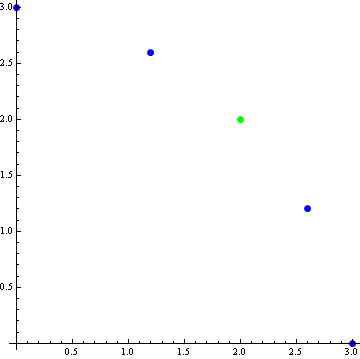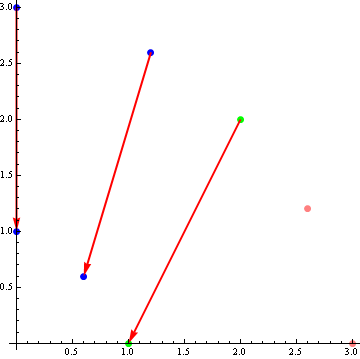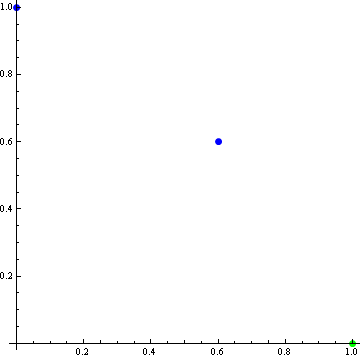# 12

Personal Blog

Back in the old days, when people were wise and the government was just, I did a post on the Nash bargaining solution for two player games. Here each player has their own utility function and they're choosing amongst joint options, and trying to bargain to find the best one. What was nice about this solution is that it is independent of irrelevant alternatives (IIA): once you've found the best solution, you can erase any other option, and it remains the best.

In order to do that, the Nash bargaining solution makes use of a "disagreement point", a special point that provides a zero to both utilities. This seems - and is - ugly. Can we preserve IIA without this clunky disagreement point?

By the title of the this post, you may have guessed that we can't. Specifically, assume the outcome is symmetric across both players (i.e. permuting the two utility functions preserves the outcome choice), the outcome is Pareto-optimal (any change will reduce the utility of at least one player) and there is no outside canonical choices for the utility functions (no special scales, no zeroes, no disagreement points). Then IIA must fail. It fails under weaker conditions as well, but the above lead to an easy picture-proof. And picture proofs are nice.

So assume there are five possible choices, whose utility values for the two players are (0, 3), (1.2, 2.6), (2, 2), (2.6, 1.2), (3, 0). These are graphed here:The choice set is symmetric and the green point (2, 2) is Pareto-optimal and on the axis of symmetry. Hence by the assumptions, the green point must be the outcome chosen. Now further assume IIA, and we will derive a contradiction.

First, by IIA, we can erase the losing points (2.6, 1.2) and (3, 0). Then we can rescale the utility functions: the utility function graphed on the x axis is divided by two, while the utility graphed on the y axis has 2 subtracted from it. These changes are illustrated here:This results in a final setup of (0, 1), (0.6, 0.6) and (1, 0):But this is obviously wrong: symmetry implies the correct outcome should be the blue point (0.6, 0.6), not the green (1, 0) which was the outcome before we removed the "irrelevant" extra points. We have derived a contradiction, and IIA must fall.

# 12

New Comment

In your initial argument you use symmetry. Then you introduce a scaling that breaks the symmetry. I think you are misapplying the axiom of no canonical scale. This is fine for one utility function. But if you're going to say that such-and-such a point is on an axis of symmetry of two different utility functions, then in effect you have introduced a constraint: Applying symmetry-breaking scalings will now give you nonsensical results - as you indeed find. But the problem is with the initial assumption of symmetry followed by the deliberate breaking of symmetry, not with IIA.

The rescalings have no mathematical meaning. They are for pictorial understanding only. Utility functions do not have any intrinsic scales at all. And so there isn't such a thing as a "rescaling" - the utility function is the class of functions related by positive affine transformations, so rescalings do not change this class.

So what does symmetry mean then? Well, it means that if we interchange the two (classes of) utility functions, we end up in the same situation. This is equivalent with "we can choose scales so that the picture we draw with those scales is symmetric", hence my pictures.

Suppose the axes are not showing the utilities of independent agents, but a single agent's utility from two arguments, say ice cream and sleep. We see that the (2,2) point with its total utility of 4 is preferred by this single agent. Halve the utility from ice cream; the agent now prefers the former (1.2, 2.6) point, which has been transformed to a (0.6, 2.6) point with a total utility of 3.2. Clearly then this is not the same utility function. This demonstrates that scaling one axis in a combined utility function is not an affine transformation: It does not preserve ordering.

I don't see why you expect two independant agents with their own coherent utility functions to have a coherent combined utility function, and it seems that the point of Stuart's argument is to show that there are cases where they can't.

Ok; I thought of a better way to phrase Stuart's point. Suppose there are five alternatives, and I rank them 1-2-3-4-5, but you rank them 5-4-3-2-1. If we are equal in power we will compromise on 3. (Well... given some simplifying assumptions, anyway. It's quite possible that you are almost indifferent between 2 and 3, but I care a lot about that gap. If so, even if we are equal in power I will likely commit a lot more resources to the fight, and drag the compromise up to 2.) But if the available options had been 1, 2, and 3, we would instead have compromised on 2. This demonstrates that removing options changes the outcome.

However, I think there is a problem with carrying the "irrelevant alternatives" axiom into a two-agent problem. If I have A>B>C, then I should choose A whether or not C is an option; fine. But this needn't be true of problems with multiple agents, because that phrase "we will compromise on" is hiding rather a lot of complexity that doesn't have anything to do with utility functions, per se. Options 4 and 5 are not, in fact, irrelevant; they are bargaining chips. Removing one side's bargaining chips breaks the symmetry; it is equivalent to giving the other side more power. Suppose I had left the options as they were, but specified that the agent whose utility is on the y axis suddenly gets a lot more bargaining power; would we then expect the decision to be option 3? Surely not. And this is exactly what is accomplished by asymmetrically removing options.

The problem rises from breaking the game-theoretic symmetry and asserting that only the utility symmetry is important.

The most common formulation of IIA precisely assumes that there is no such thing as "bargaining chips". So yes, you could rewrite the point of my post as: any symmetric bargaining solution will have bargaining chips.

The point is that if the transformation that Stuart uses were applied to a single agent, it would convert a coherent utility function into an incoherent one; therefore it cannot demonstrate anything about the incoherence of combined utility functions. It is too general - in fact, it is a Fully General Counterargument to the existence of utility functions with more than one input. It could well be the case that independent agents cannot have a coherent combined utility function, but this argument does not demonstrate it unless you also wish to assert that single-agent utility functions cannot consist of linear additions of sub-utilities.

in fact, it is a Fully General Counterargument to the existence of utility functions with more than one input

No, it's not, because it is not even talking about a utility function with more than one input. It is talking about two completely seperate utility functions. A single utility function with multiple inputs has to include a scaling between the inputs and therefore is not described by Stuart's argument, which exploits the lack of such scaling between two seperate utility functions.

Why does IIA allow you to rescale the two utility axes using different scaling functions?

I think this is a feature of utility functions rather than the IIA assumption; they are unique up to a scaling and offset (positive affine transformation). See this wikipedia article for more. The intuition is that, adding an offset to the whole function, or rescaling it does change the relative comparisons of different points on that function and thus doesn't change the choices for an agent with that utility function.

Assumption that there is no canonical scales for the utility functions.

How does having a "disagreement point" prevent this same scaling trick? It seems that the issue is more-so with the ability to arbitrarily rescale using a different scaling function for each axis...

The disagreement point gives origins to both utilities, and prevents translations. It turns out that IIA can apply if only scalings are allowed, even different scalings (see Nash bargaining in my other post)

Basically this says that decision symmetry and IIA are incompatible because the definition of "symmetry" depends "irrelevant alternatives". Yes?

In a way.

But as I said, you don't actually need symmetry to break IIA (non-dictatorship should be enough); it's just that there is an easy picture proof for symmetry.

[-][anonymous]11y 0

Stuart_Armstrong, I figured I should try and lay out the math without pictures to check for my understanding. Am I doing this correctly?

Here are the choices, which are Ordinally Symmetric:

(0, 3), (1.2, 2.6), (2, 2), (2.6, 1.2), (3, 0).

Here is those choices expressed as a preference order (I.e, 1st is most preferred, 5th is least preferred, units are irrelevant.)

(5th, 1st), (4th, 2nd), (3rd,3rd), (2nd,4th), (1st,5th).

All points are Pareto Optimal, so Symmetry is used to decide that among these:

(0, 3), (1.2, 2.6), (2, 2), (2.6, 1.2), (3, 0).

(5th, 1st), (4th, 2nd), (3rd,3rd), (2nd,4th), (1st,5th).

The choice would be this:

(2,2)

(3rd,3rd)

Now, let's remove two irrelevant choices:

(0, 3), (1.2, 2.6), (2, 2)

(5th, 1st), (4th, 2nd), (3rd,3rd),

Now, we have to rescale one of the preference scales: (I think? Having a third, fourth, and fifth preferred choice among three choices doesn't seem to make any sense.)

(0, 3), (1.2, 2.6), (2, 2)

(3rd, 1st), (2nd, 2nd), (1st,3rd)

And, then we rescale this Ordinally, keeping them in the same preference order: (x=x/2, y=y-2)

(0, 1), (.6, .6), (1, 0)

(3rd, 1st), (2nd, 2nd), (1st,3rd)

And now the symmetric choice is (.6, .6), which it wasn't before.

Whereas if we don't remove the irrelevant alternatives.

(0, 3), (1.2, 2.6), (2, 2), (2.6, 1.2), (3, 0).

(5th, 1st), (4th, 2nd), (3rd,3rd), (2nd,4th), (1st,5th).

And then we rescale them (x=x/2, y=y-2)

(0, 1), (.6, .6), (1, 0), (1.3, -.8), (1.5, -2).

(5th, 1st), (4th, 2nd), (3rd,3rd), (2nd,4th), (1st,5th).

Now they aren't Ordinally symmetric. Only removing those points earlier allowed it to be Ordinally symmetric again after the rescale.

Does this correctly capture the point of the above pictures?

Nearly. But I used more than an ordinal symmetry to be able to actually select the points I wanted (ordinally, "choice 3" is the same as "50% between choices 1 and 5", "50% between choices 2 and 4" and so on; I used the stronger symmetry to be able to choose option 3).

But this is obviously wrong: symmetry implies the correct outcome should be the blue point (0.6, 0.6), not the green (1, 0) which was the outcome before we removed the "irrelevant" extra points. We have derived a contradiction, and IIA must fall.

Isn't the correct outcome (1.0, 0), not (.6, .6)? If you rescale the utility functions and your decision changes, you aren't using the utility functions correctly.

That is, as RolfAndreassen points out, you can't use symmetry to choose the 'correct' outcome if you use a symmetry-breaking transformation.

If you rescale the utility functions and your decision changes, you aren't using the utility functions correctly.

Precisely. So the error arrived when we removed the extra points and IIA implied the decision didn't change.

That is, as RolfAndreassen points out, you can't use symmetry to choose the 'correct' outcome if you use a symmetry-breaking transformation.

There is no transformation. Scalings are aretefacts of how we represent the utility functions. They have no intrinsic meaning. So I have not been using a "symmetry-braking transformation" - I haven't used a transformation at all, just a different way of drawing the exact same situation.

Precisely. So the error arrived when we removed the extra points and IIA implied the decision didn't change.

What? (2,2) is still on the axis of symmetry, regardless of whether or not the point (2.6, 1.2) exists or not, and so if they select that point because of symmetry, they will continue to do so, regardless of the existence or nonexistence of irrelevant alternatives.

Scalings are aretefacts of how we represent the utility functions. They have no intrinsic meaning. So I have not been using a "symmetry-braking transformation" - I haven't used a transformation at all, just a different way of drawing the exact same situation.

Not in this problem, because you've set it up so the axis of symmetry is used for decision-making. If you do the scaling to both the points and the axis of symmetry, then you get the correct answer- (1.0, 0) - because it lies on the line y=2x-2, which is the new axis of symmetry. By only scaling part of the problem, you perform a transformation.

 Thinking about this more, I think I've modified my position somewhat: you have two assumptions, first that the outcomes are symmetric and second that there are no canonical choices for utility functions. Those don't look like they play well together- if outcome (0,3) is symmetric with outcome (3,0), and then by changing your choice of utility function you can make (0,3) symmetric with (2,2), then you have a serious problem. If you fulfill the symmetry assumption by restricting IIA to removing pairs of symmetric points, the crisis is averted.

[edit2] There's a more general point that should be mentioned- whenever you have a decisionmaker whose decision depends on relative outcome merits, then IIA either breaks or is limited severely. "Irrelevant" needs to be understood not as "outcomes I didn't choose" but "outcomes which didn't impact my choice." When your rule is "pick the second best," then both the best and second best are relevant alternatives, even though you only picked one. In a bargaining game without a frame, the only way to judge outcomes is by their relative merits- and so you get weak or broken IIA.

But in this particular problem, the symmetry assumption throws a wrench into that general point, because now there is a frame- the symmetric lattice, and the implied axis of symmetry- and so there's an objective criterion rather than simply relative criteria.

What? (2,2) is still on the axis of symmetry, regardless of whether or not the point (2.6, 1.2) exists or not, and so if they select that point because of symmetry, they will continue to do so, regardless of the existence or nonexistence of irrelevant alternatives.

The axis of symmetry is a property of the figure (in this case, the set of points), not of the axis. In fact, ignore the axis: they don't exist, only their directions have mathematical meaning (neither their scale nor their points of origin mean anything, because the affine transformations of the utility functions will shift those).

Right- let's just call the points A, B, C, -B, and -A. C is going to be the middle of that set, even if you remove (B and -B) and/or (A and -A). When you remove (-B and -A), you've broken the symmetry of the set, even though the set has new symmetry by virtue of there being a new middle point.

If you're postulating a (much much) weaker version of IIA saying something like "if you remove symmetric irrelevant points from a symmetric set, the outcome doesn't change" then you'd be right. But IIA does not require that symmetry be preserved.

Yep, I agree that strong IIA of "if x is chosen from T, and S is a subset of T, then x is chosen from S" doesn't apply if preferences are based on the relative merits of x rather than the individual merits of x. That statement seems obviously true on its own, and so I think the picture proof of this particular example detracts more than it adds, because there is a natural weak IIA here.